# Maple CTF 2022Figure 1. Maple-chan on a custom-themed CTFd platform.

We hosted our inaugural CTF event last weekend, attracting over 1000 teams and 5000 users from around the world. Compared to the local university-wide competition that we ran earlier in the year, Maple CTF came with more unique and creative challenges. In this post, I’ll share solutions to the 3 cryptography challenges that I wrote, as well as some organizational tidbits from organizing the event.

# jwt [79 solves]

I just learned about JWT tokens, but everyone’s using HS256 and RS256. Why not show some love for elliptic curves?

Files can be found here.

After seeing the majority of JWT-based CTF challenges rely on vulnerabilities in HS256 and RS256 encryption, I created a beginner-friendly challenge demonstrating that alternative asymmetric algorithms such as ECC can also be used for signatures and verification.

class ES256:
def __init__(self):
self.G = secp256k1.G
self.order = secp256k1.q
self.private = private
self.public = self.G * self.private

def _sign(self, msg):
z = sha256(msg.encode()).digest()
k = self.private

z = bl(z)

r = (k * self.G).x
s = inverse(k, self.order) * (z + r * self.private) % self.order

return r, s

def _verify(self, r, s, msg):
if not (1 <= r < self.order and 1 <= s < self.order):
return False

z = sha256(msg.encode()).digest()
z = bl(z)

u1 = z * inverse(s, self.order) % self.order
u2 = r * inverse(s, self.order) % self.order

p = u1 * self.G + u2 * self.public

return r == p.x

# return true if the token signature matches the data
def verify(self, data, signature):
r = int.from_bytes(signature[:32], "little")
s = int.from_bytes(signature[32:], "little")

return self._verify(r, s, data)

# return the signed message and update private/public
def sign(self, data):
...

# return the decoded token as a JSON object
def decode(self, token):
...


To get the flag, you have to forge a token and log in as the admin account.

## Solution

The solution exploits the common mistake of ECDSA nonce reuse. In this case, the nonce is the same as the private key, meaning that an attacker can easily solve the ECDSA equation:

$$s = k^{-1}(z + rd)$$ $$s = d^{-1}(z + rd)$$ $$s-r = d^{-1}z$$ $$d = z / (s-r)$$

Implementation:

# login with any username and copy the jwt token

r = int.from_bytes(signature[:32], "little")
s = int.from_bytes(signature[32:], "little")

G = secp256k1.G
order = secp256k1.q
msg = b'eyJhbGciOiJFUzI1NiIsInR5cCI6IkpXVCJ9.eyJ1c2VyIjoiYWJjZCJ9'
z = sha256(msg).digest()
z = bl(z)

# find the private key
private = inverse((s - r) * inverse(z, order), order)
print(private)

# forge a new token
from jwt import ES256
es = ES256(private)


## Unintended!

As per the RFC for JWT, the data to be signed should be stripped of all spaces. Unfortunately, I only removed these spaces after the user registered, meaning that they could create an account like:

username: ad  mi n


Since the JWT implementation recognizes that username as admin, the token they received would be valid for a flag.

# Spiral-baby [57 solves]

Spiraling into confusion.. Note: this is an easier version of the Spiral challenge, the only difference is the number of encryption rounds.

Files can be found here.

For this challenge, I implemented a custom block cipher based upon AES and Square, with the 4 operations:

2. substitute
3. rotate (rotate the matrix clockwise)
4. mix (multiply by a coefficient matrix)

Users are given an encryption oracle, as well as the encrypted flag. In the “baby” version, there’s only 1 round of encryption.

class Spiral:
def __init__(self, key, rounds=4):
self.rounds = rounds
self.keys = [bytes2matrix(key)]
self.BLOCK_SIZE = 16

for i in range(rounds):
self.keys.append(spiralLeft(self.keys[-1]))

def encrypt(self, plaintext):
if len(plaintext) % self.BLOCK_SIZE != 0:
padding = self.BLOCK_SIZE - len(plaintext) % self.BLOCK_SIZE

ciphertext = b""
for i in range(0, len(plaintext), 16):
ciphertext += self.encrypt_block(plaintext[i : i + 16])
return ciphertext

def encrypt_block(self, plaintext):
self.state = bytes2matrix(plaintext)

for i in range(1, self.rounds):
self.substitute()
self.rotate()
self.mix()

self.substitute()
self.rotate()

return matrix2bytes(self.state)

for i in range(4):
for j in range(4):
self.state[i][j] = (self.state[i][j] + self.keys[idx][i][j]) % 255

def substitute(self):
for i in range(4):
for j in range(4):
self.state[i][j] = SBOX[self.state[i][j]]

def rotate(self):
self.state = spiralRight(self.state)

def mix(self):
out = [[0 for _ in range(4)] for _ in range(4)]
for i in range(4):
for j in range(4):
for k in range(4):
out[i][j] += SPIRAL[i][k] * self.state[k][j]
out[i][j] %= 255

self.state = out


## Solution

We can trace out each operation:

2. substitute()
3. rotate()

It turns out that we don’t even have to worry about the mix operation! We can follow what happens to a specific byte of the plaintext: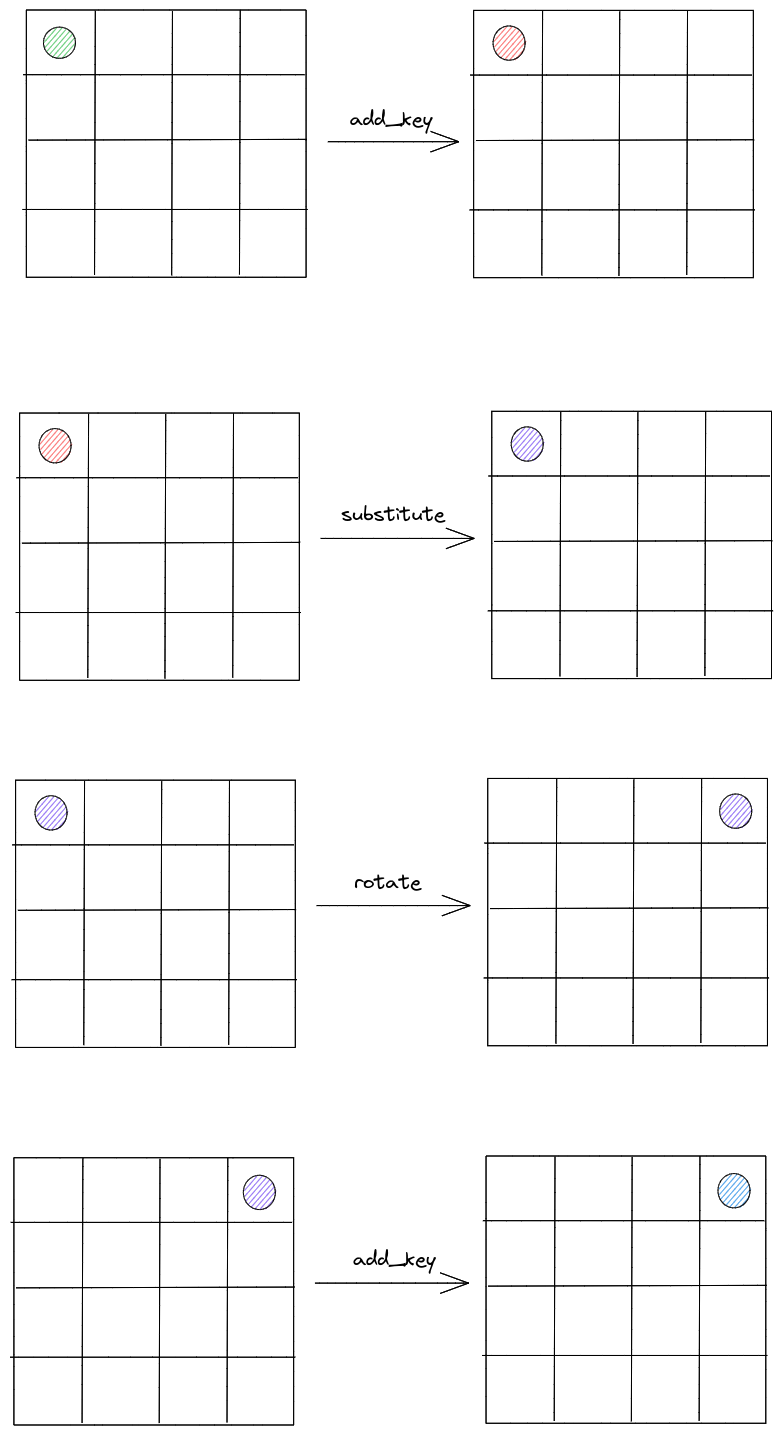Consider the first byte of the plaintext (top-left corner). It first undergoes the add_key operation with an unknown key byte (red), then substitution with a known S-Box (purple). Rotate then changes the position of that key byte, followed by a second add_key operation (blue).

Let’s call the first byte of the key $k_1$ and the other key byte $k_2$. If we know $k_1$, we can calculate the intermediate states up to the final add_key. Since we also know the final ciphertext, we can easily recover $k_2$ by reversing the last add_key operation.

Based on this, our attack to recover the first byte is as follows:

1. Encrypt a handful of plaintexts with differing first bytes
1. Guess a value for $k_1$ (out of 256 possibilities)
1. Calculate $k_2$ for each of the differing plaintexts
1. If our guess of $k_1$ is correct, then all of the $k_2$ values should be the same. At this point, we have found $k_1$ and $k_2$
1. If $k_2$ differs between ciphertexts, repeat (2) with a new value of $k_1$.

We can repeat this for all 16 bytes to recover the key, each time using a set of plaintexts with different values in the position we want.

# Spiral [9 solves]

This is the same challenge as Spiral-baby, but now with 4 rounds of encryption! Our previous attack no longer works because of the mix operation, which provides the primary source of diffusion. It’s similar to the original AES mixColumns, but instead we perform matrix multiplication in the integers modulo 255.

Files can be found here.

## Solution

The intended solution is based on the Square attack, also known as integral cryptanalysis. The central idea is to encrypt a set of plaintexts, where bytes in a specific position are varied and the other bytes are held constant. By examining how properties of this set change through each round of encryption, we can recover the key.

This attack breaks up to 6-rounds of AES, although cryptanalysis beyond 5 rounds requires immense computational power. NCC Group’s Block Breakers website has a great section on the square attack - I would highly recommend checking that out and applying that to this problem.

The key idea of the square attack is to choose an index and make it take on all 256 byte values. We call this an active index.

When a plaintext undergoes the add_key or substitute rounds, the index remains an active index because both of these operations are bijective.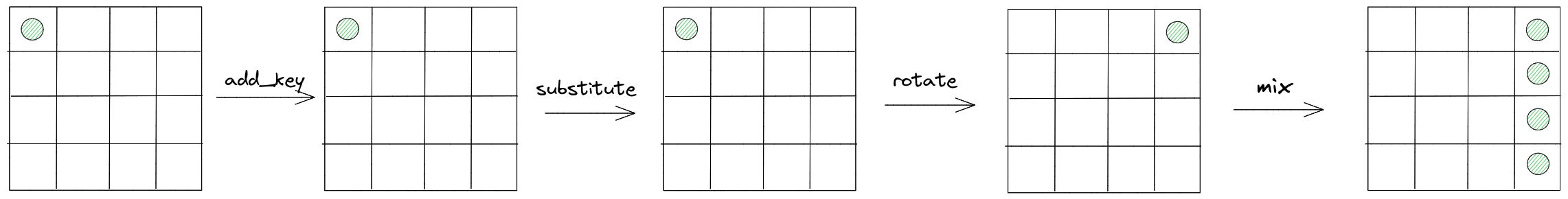Let’s demonstrate how this works when the first byte is the active index. In the diagram above, we’re tracing through each step of first encryption round. Here, green denotes an active index. Notice how the mix operation forces the entire column to be active if a single index is active - this can be shown by examining the matrix multiplication operation.

Now, let’s look at what happens after the second round of encryption.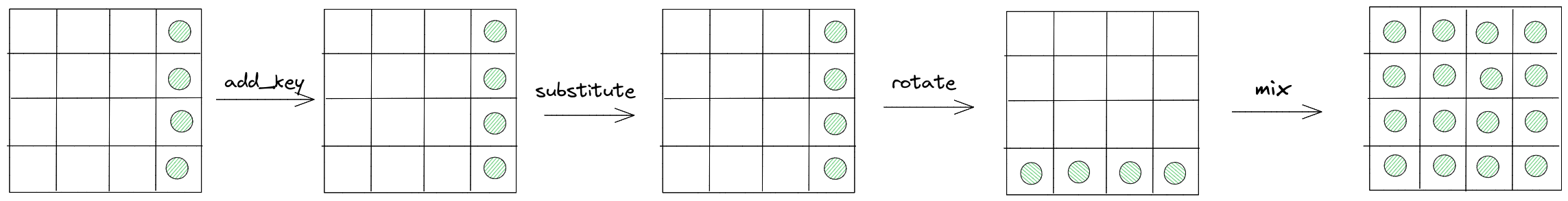So far, all of the operations have created new active indices. But, things start to get interesting after the third round of encryption. What will happen to mix if all of the bytes are already active bytes?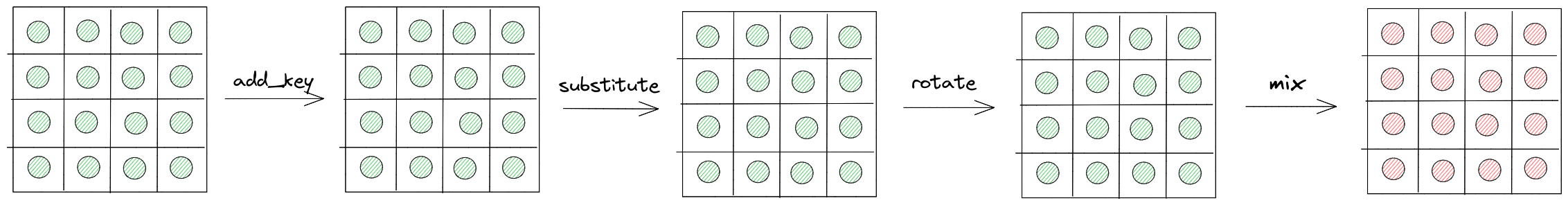What happens in this round’s mix operation? Let’s consider the first column, which is dotted with the vector $[1, 19, 22, 23]$ to produce the byte at position 0.

Let $b_j$ be the first byte of the $jth$ plaintext after mix, and let $a_{i, j}$ represent the byte at position $i$ for the $j$th plaintext before mix. So, the first column of the first plaintext would be represented by $a_{0, 0}, a_{4, 0}, a_{8, 0}, a_{12, 0}$. Then, we have the following:

$$1a_{0, 0} + 19a_{4, 0} + 22a_{8, 0} + 23a_{12, 0} \equiv b_0 \mod 255$$ $$1a_{0, 1} + 19a_{4, 1} + 22a_{8, 1} + 23a_{12, 0} \equiv b_1 \mod 255$$ $$\dots$$ $$1a_{0, 255} + 19a_{4, 255} + 22a_{8, 255} + 23a_{12, 255} \equiv b_{255} \mod 255$$

If we compute $b_0 + b_1 + \dots + b_{255}$, we get:

$$1(a_{0, 0} + \dots a_{0, 255}) + 19(a_{4, 0} + \dots a_{4, 255}) + 22(a_{8, 0} + \dots a_{8, 255}) + 23(a_{12, 0} + \dots a_{12, 255})$$

Since $a_{0, 0}, a_{4, 0}, a_{8, 0}, a_{12, 0}$ are active indices that take on all 255 values, their sum modulo 255 is 0. Therefore, the above expression reduces to:

$$1 \cdot 0 + 19 \cdot 0 + 22 \cdot 0 + 23 \cdot 0 \equiv 0 \mod 255$$

In other words, we’ve found a relationship that holds after the mix columns operation! If we pick any position, the sum of the bytes in that position must be divisible by 255. You can easily verify this yourself by following these steps with the actual Spiral implementation.

Finally, the last round looks like: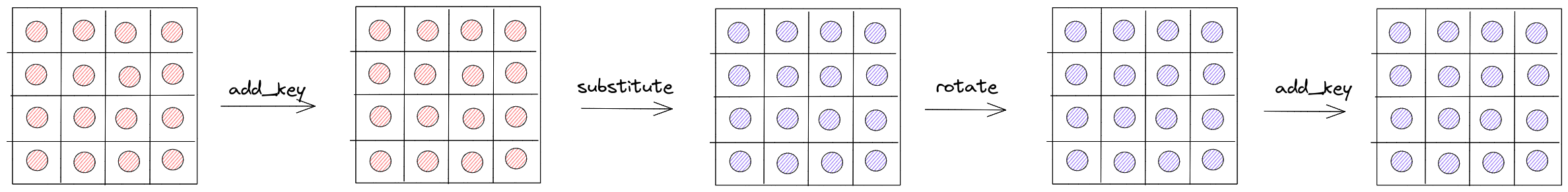The add_key step will preserve the invariant we found above, but since substitute is non-linear, our observations break down after that step. However, we can apply a technique commonly used in cryptanalysis: guessing the last round’s key bytes.

Imagine we knew the 4th byte byte of the last round’s key. Then, we could step back through add_key, rotate (now the byte corresponds to the first position), and substitute.

We can tell if our guess is correct if the sum of the bytes in that position over all plaintexts is divisible by 255. This might still be true for bytes that are not the actual key bytes, but we can encrypt a different set of plaintexts with the same active index to eliminate false positives.

So, our algorithm boils down to:

1. Encrypt 256 plaintexts with an active index for each of the 16 positions
1. For each position of the last round key, guess a byte
1. Reverse the final round add_key, rotate, and substitute steps
1. Compute the sum of the bytes in the inverse-rotated position
1. If the sum is divisible by 255, add the byte to a pool of candidates
1. Repeat step 1 until there is only one possible key byte for a specific position.

At this point, we’ve found the key for the last round! Since the round keys are simply rotated clockwise from each other, the 4th round key is the same as the original key. All that’s left is to implement the inverse of the various steps:

• add_key -> subtract_key
• rotate -> counterclockwise-rotate
• substitute -> inv_substitute
• mix -> inv_mix (multiply by inverse of coefficient matrix)

Congrats! Here’s the described attack in code:

for pos in range(16):
possible = set([i for i in range(255)])

while len(possible) > 1:
plaintexts = []
ciphertexts = []

pt = [i for i in range(16)]
random.shuffle(pt)

# encrypt the 255 plaintexts together for increased speed
big_pt = b''
for i in range(255):
pt[pos] = i
big_pt += bytes(pt)

big_ct = bytes.fromhex(query(big_pt).decode())

for i in range(0, len(big_ct), 16):
plaintexts.append(big_pt[i:i+16])
ciphertexts.append(big_ct[i:i+16])

correct = set()
for keyGuess in range(255):
total = 0
for pt, ct in zip(plaintexts, ciphertexts):
# trace through the last round's steps
byte = (ct[pos] - keyGuess) % 255
byte = INV_SBOX[byte]
total += byte

# the byte is potentially correct if the sum over all ciphertexts is divisible by 255
if total % 255 == 0:

possible = possible.intersection(correct)

assert len(possible) == 1
key.append(possible.pop())

print(bytes(key))


Additional solve files, including decryption, can be found here.

# CTF Organization

## Pre-CTF

After our success at DEFCON CTF, we realized we had 2 weeks left until our CTF started. We needed to get things moving - publish the website, test and deploy challenges, etc. Our team was divided into challenge authors, infra, and frontend, with some overlap in-between.

Luckily, our 1-person frontend team (credits to @kewbish) made a wonderful reskin of the default CTFd hosting platform. We set up mailgun to automatically send confirmation emails for registration, and we were ready to announce Maple CTF!

Some of our team went down with COVID from DEFCON the earlier week, so we made the most out of the situation and pushed out our challenges ASAP.

Although we planned out our challenge ideas months in advance, we still needed time to implement them. Our infrastructure was managed with Kubernetes on Google Cloud (our infra sponsor), set up by Benson - he has a great writeup describing the infrastructure in detail.

## Mid-CTF

Just like with almost every other CTF, our CTFd started freezing for the first ~10 minutes. We initially had a monolithic instance with 65 gunicorn workers, but we quickly scaled it to 3 different pods.

After a few hours, the top teams began inching closer toward a full-solve, with only a few hard challenges remaining. Because of our fixed 24-hour release schedule, this would mean that new challenges might be released after they had already full-solved everything - not what we wanted!

Later that night, a team reported to us an “unintended” solution for one of our reversing challenges, vm. Although the goal of the challenge was to reverse the steps of a VM written in Verilog, we had accidentally left the flag in hex directly in the files we distributed. At this point, we had a few options:

• Keep the challenge as is
• Deploy a fixed challenge and remove all points for vm
• Deploy a fixed challenge and decrease the scoring for vm

We decided that the latter option was the most fair, leading to the creation of vm-v2.

Our OSINT challenge turned out to be quite problematic. The intended solution was to find a user’s twitter account and perform manual barcode recovery on an image, but since we hadn’t specified the scope of the competition, users began submitting random names from all over the internet. Someone in the Discord server even named themselves after the fictional OSINT character, leading to countless submissions of their real name: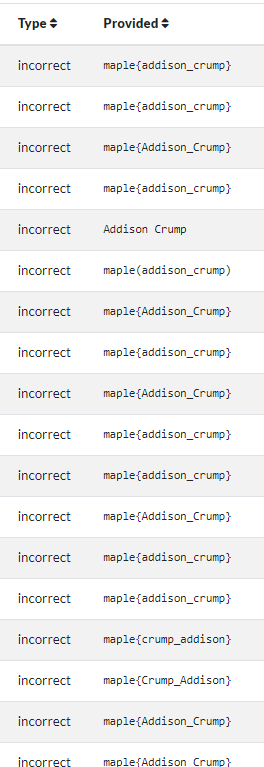In the end, we released a few hints regarding the OSINT challenge which better specified the scope of the solution.

Everything went pretty smoothly after that, including the 24-hour release of challenges. Our ticketing system based off the Discord Tickets bot worked very well, with over 300 questions successfully resolved.

## Post-CTF

With the conclusion of the CTF, we announced the winners and additional writeup prizes. Additionally, we hosted an internal retrospective, looking at what went well and what didn’t. Some lessons learned:

• Keep the infrastructure and challenge dev teams in sync, any requirements should be clearly communicated.
• At least 2 weeks for testing, looking out for unintended solutions and bugs.
• No OSINT challenges.
• Standardized Dockerfile configurations for different types of challenges.
• Flexible release schedule instead of fixed 24-hour release.
• Challenge devs should be familiar with kubectl basics to debug their own challenges if something goes wrong.

# Closing Remarks

Overall, hosting Maple CTF was a blast! We never expected so many teams to play in our competition, and we’re grateful to everyone who tried out our challenges. We’re planning to host another local CTF next year, followed by Maple CTF 2023 in the autumn. See you there! 🍁🥓